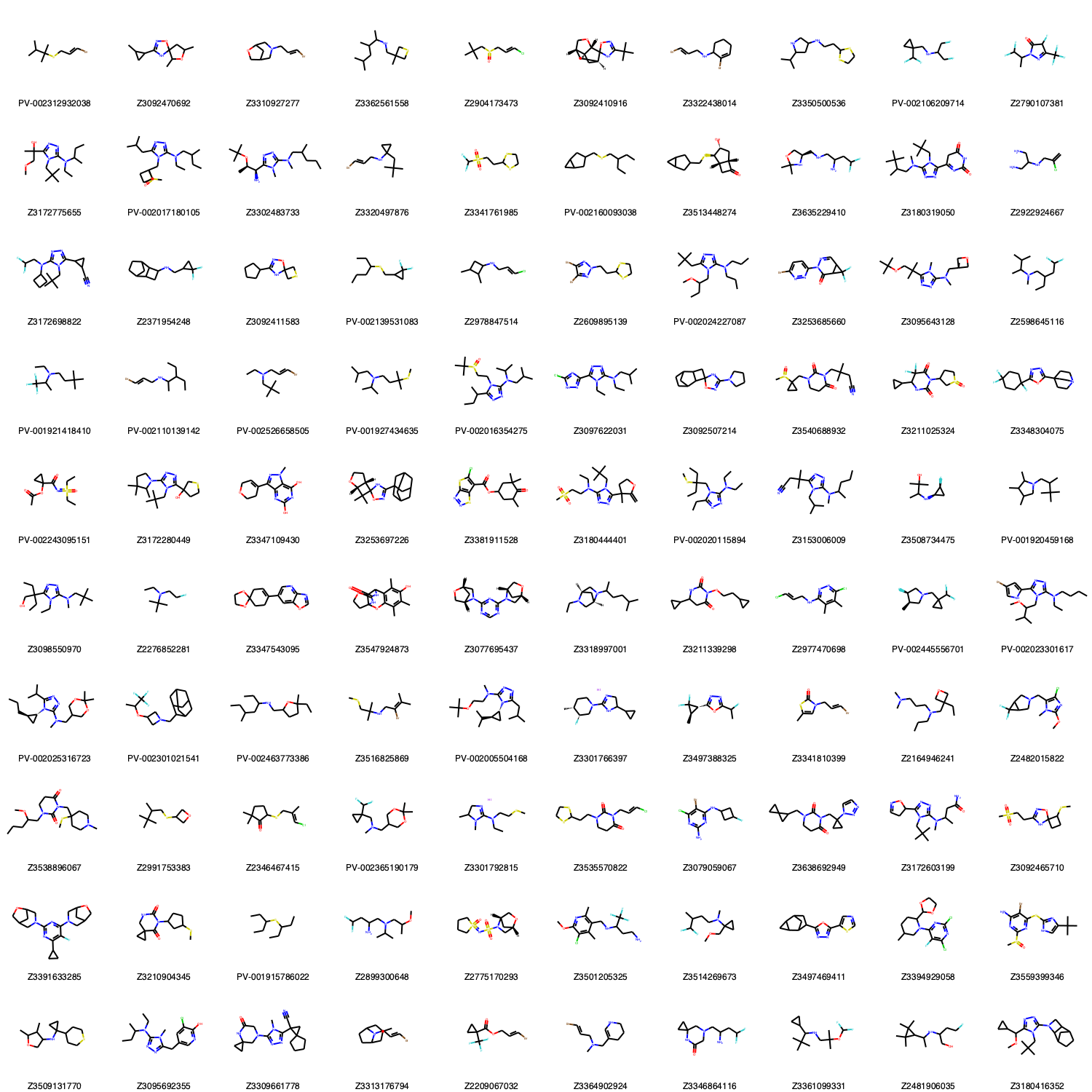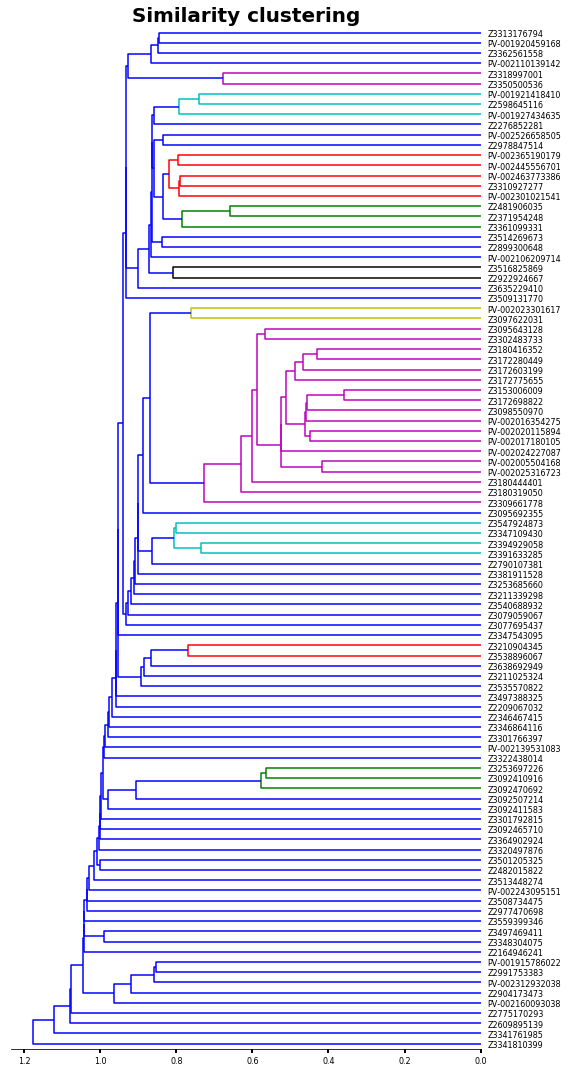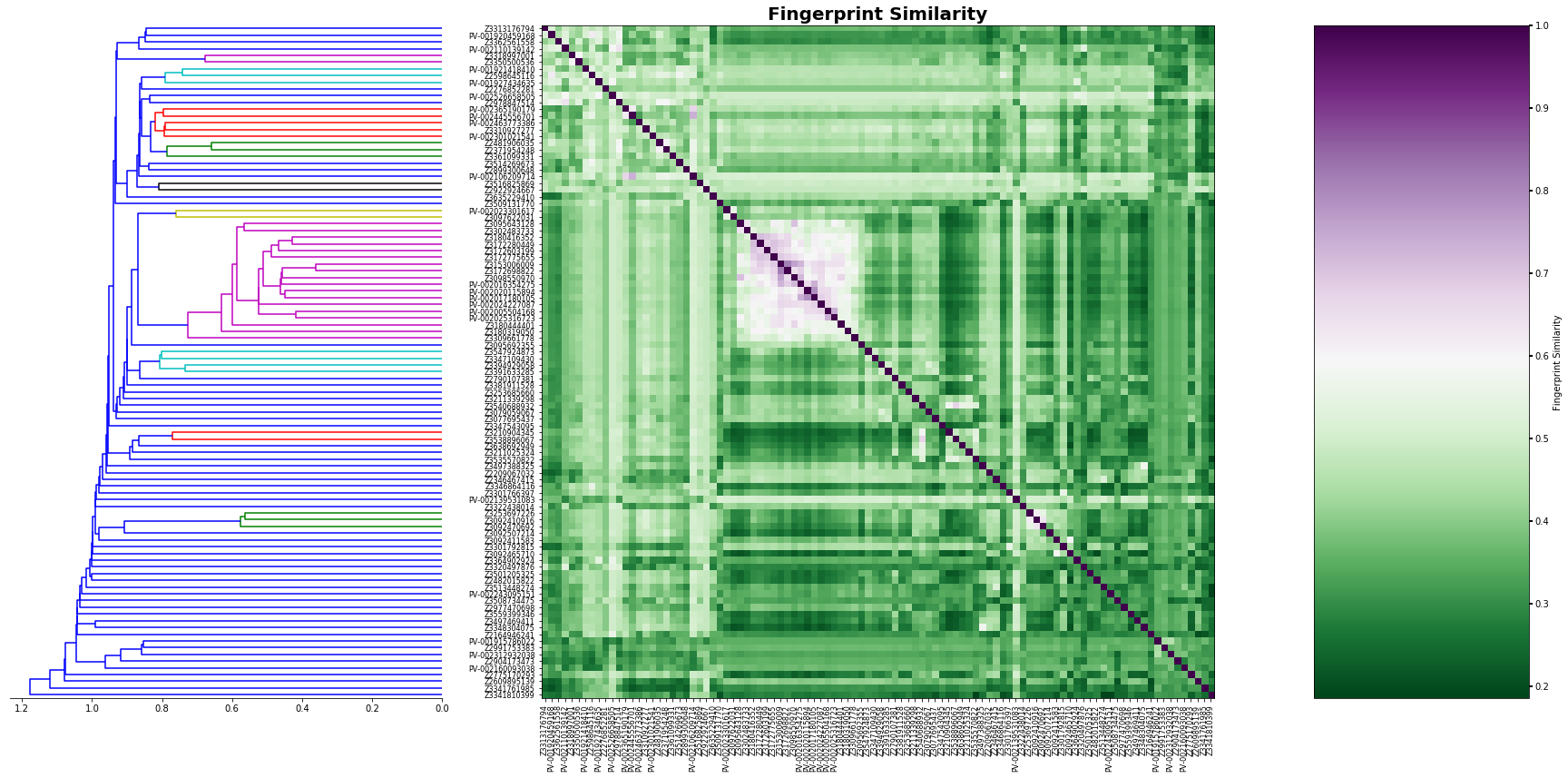# Similarity analysis of compound databases¶

In this chem-workflow, I will show you a strategy to calculate the similarity of a molecule database in a straightforward manner.

For this purpose, I will use a fraction of the REAL Compound Library of Enamine. You can read more about the database here.

Despite I will use a SMILES file, this analysis can be performed from SDF codes of molecules or any other format in the same way. Just be sure of using the appropriate supplier for loading your molecules into RDKIT.

## Importing the libraries we need¶

In :
import os
import pandas as pd
import numpy as np
import matplotlib.pyplot as plt
from matplotlib import gridspec

from rdkit import Chem, DataStructs
from rdkit.Chem.Fingerprints import FingerprintMols
from rdkit.Chem import Draw

# All we need for clustering


The library contains more than 8 000 000 SMILES codes (size 542.8 M). But we can read the .smiles file as a text file to keep the fist 100 molecules.

In :
# The result of this code will be:
working_library=[]
with open('Enamine_REAL_diversity.smiles','r') as file:
for index,line in enumerate(file):
if 0<index<=1: # Kee the fist smile code as example
print (index, line.split())

1 ['CC(C)C(C)(C)SCC=CBr', 'PV-002312932038']


The result of the above cell is a list which first element (0) is the SMILES codes of the molecule, and the second element (1), the name.

So, we can use the same code to convert SMILES codes to RDKIT molecules.

In :
working_library=[]
with open('Enamine_REAL_diversity.smiles','r') as file:
for index,line in enumerate(file):
if 0<index<=100: # Molecules we want (0 is omitted because the fist line (0) of file is the header, not SMILES code)
mol=Chem.MolFromSmiles(line.split()) # Converting SMILES codes into rdkit mol
mol.SetProp('_Name',line.split()) # Adding the name for each molecule
working_library.append(mol)


Now we have a list of 100 RDKIT type molecules to perform our similarity analysis.

Let´s draw them to see what is inside.

In :
Draw.MolsToGridImage(working_library,molsPerRow=10,subImgSize=(150,150),legends=[mol.GetProp('_Name') for mol in working_library])

Out:## Getting the fingerprints for comparison¶

For fingerprint similarity analysis, we first need to get the fingerprints for each molecule.

For such purpose we type:

In :
fps= [FingerprintMols.FingerprintMol(mol) for mol in working_library]


As result we have n fingerprints as n molecules:

In :
print(len(working_library))
print(len(fps))

100
100


And we can get the similarity for each pair of molecules.

For instance mol_1 as reference and mol_2 as target.

In :
DataStructs.FingerprintSimilarity(fps,fps)

Out:
0.3438735177865613

Following the above example, we can construct all vs all analysis to see the similarity within our database (Something similar can be found in another Chem-workflow of this site -click here to see that entry-)

In :
size=len(working_library)
hmap=np.empty(shape=(size,size))
table=pd.DataFrame()
for index, i in enumerate(fps):
for jndex, j in enumerate(fps):
similarity=DataStructs.FingerprintSimilarity(i,j)
hmap[index,jndex]=similarity
table.loc[working_library[index].GetProp('_Name'),working_library[jndex].GetProp('_Name')]=similarity


Let´s take a look to the similarity values

In :
table.head(10) # just the first 10 values due to our table in 100 x 100

Out:
PV-002312932038 Z3092470692 Z3310927277 Z3362561558 Z2904173473 Z3092410916 Z3322438014 Z3350500536 PV-002106209714 Z2790107381 ... Z3509131770 Z3095692355 Z3309661778 Z3313176794 Z2209067032 Z3364902924 Z3346864116 Z3361099331 Z2481906035 Z3180416352
PV-002312932038 1.000000 0.343874 0.342995 0.339394 0.379845 0.342520 0.369792 0.319588 0.282353 0.331984 ... 0.324675 0.335938 0.339844 0.324324 0.317308 0.333333 0.294931 0.312821 0.303922 0.335938
Z3092470692 0.343874 1.000000 0.512712 0.307036 0.349020 0.635063 0.428270 0.411765 0.505882 0.395076 ... 0.413333 0.257565 0.346515 0.377399 0.453975 0.356688 0.291557 0.410638 0.434599 0.350902
Z3310927277 0.342995 0.512712 1.000000 0.313480 0.362319 0.510204 0.349162 0.369628 0.497674 0.445676 ... 0.528947 0.474227 0.494071 0.430380 0.361413 0.369231 0.392105 0.416918 0.380682 0.485030
Z3362561558 0.339394 0.307036 0.313480 1.000000 0.315789 0.317526 0.326389 0.508000 0.456044 0.308789 ... 0.371429 0.306383 0.315261 0.409639 0.253918 0.278810 0.300912 0.420849 0.458333 0.309572
Z2904173473 0.379845 0.349020 0.362319 0.315789 1.000000 0.358268 0.335000 0.306533 0.275862 0.348178 ... 0.365639 0.351562 0.355469 0.276923 0.305164 0.276596 0.289593 0.306533 0.330049 0.351562
Z3092410916 0.342520 0.635063 0.510204 0.317526 0.358268 1.000000 0.431772 0.424490 0.503906 0.427624 ... 0.411908 0.283253 0.384665 0.382716 0.447791 0.362705 0.291517 0.417695 0.446721 0.408696
Z3322438014 0.369792 0.428270 0.349162 0.326389 0.335000 0.431772 1.000000 0.344512 0.454976 0.372768 ... 0.360000 0.418410 0.432271 0.421233 0.307042 0.397260 0.344262 0.333333 0.340299 0.414000
Z3350500536 0.319588 0.411765 0.369628 0.508000 0.306533 0.424490 0.344512 1.000000 0.456311 0.389522 ... 0.452830 0.419831 0.430862 0.423611 0.307692 0.300000 0.428152 0.418605 0.423077 0.426829
PV-002106209714 0.282353 0.505882 0.497674 0.456044 0.275862 0.503906 0.454976 0.456311 1.000000 0.504032 ... 0.508621 0.513725 0.511719 0.423645 0.514286 0.414141 0.490826 0.538462 0.557789 0.513725
Z2790107381 0.331984 0.395076 0.445676 0.308789 0.348178 0.427624 0.372768 0.389522 0.504032 1.000000 ... 0.326648 0.424028 0.465536 0.342529 0.455172 0.360849 0.294028 0.356659 0.410959 0.444564

10 rows × 100 columns

We can cluster our compound by similarity applying a linkage hierarchical clustering (HCL) analysis (Something similar can be found in another Chem-workflow of this site -click here to see that entry-).

I won´t discuss the different methods for HCL (e.g. single, complete, average, etc). Because literature is plenty of such discussions, for example, you can check the skit-learn documentation.

## HCL clustering¶

In :
linked = linkage(hmap,'single')
labelList = [mol.GetProp('_Name') for mol in working_library]

In :
plt.figure(figsize=(8,15))

ax1=plt.subplot()
orientation='left',
labels=labelList,
distance_sort='descending',
show_leaf_counts=True)

ax1.spines['left'].set_visible(False)
ax1.spines['top'].set_visible(False)
ax1.spines['right'].set_visible(False)
plt.title('Similarity clustering',fontsize=20,weight='bold')
plt.tick_params ('both',width=2,labelsize=8)
plt.tight_layout()
plt.show()Now let´s sort our initial values by the HCL analysis.

In :
# This will give us the clusters in order as the last plot
new_data=list(reversed(o['ivl']))

# we create a new table with the order of HCL
hmap_2=np.empty(shape=(size,size))
for index,i in enumerate(new_data):
for jndex,j in enumerate(new_data):
hmap_2[index,jndex]=table.loc[i].at[j]

In :
figure= plt.figure(figsize=(30,30))
gs1 = gridspec.GridSpec(2,7)
gs1.update(wspace=0.01)
ax1 = plt.subplot(gs1[0:-1, :2])
ax1.spines['left'].set_visible(False)
ax1.spines['top'].set_visible(False)
ax1.spines['right'].set_visible(False)

ax2 = plt.subplot(gs1[0:-1,2:6])
f=ax2.imshow (hmap_2, cmap='PRGn_r', interpolation='nearest')

ax2.set_title('Fingerprint Similarity',fontsize=20,weight='bold')
ax2.set_xticks (range(len(new_data)))
ax2.set_yticks (range(len(new_data)))
ax2.set_xticklabels (new_data,rotation=90,size=8)
ax2.set_yticklabels (new_data,size=8)

ax3 = plt.subplot(gs1[0:-1,6:7])
m.set_label ('Fingerprint Similarity')

plt.tick_params ('both',width=2)
plt.plot()

Out:
[]As you can see, our approach was able to identify several clusters based on fingerprint similarity, however. RDKIT can also calculate frequent-used similarity models. For example Tanimoto, Dice, Cosine, Sokal, Russel, Kulczynski, McConnaughey, and Tversky. The implementation is very easy and can be set by doing:

similarity=DataStructs.FingerprintSimilarity(i,j, metric=DataStructs.DiceSimilarity)

where:

metric - Should be one of the different methods described above.